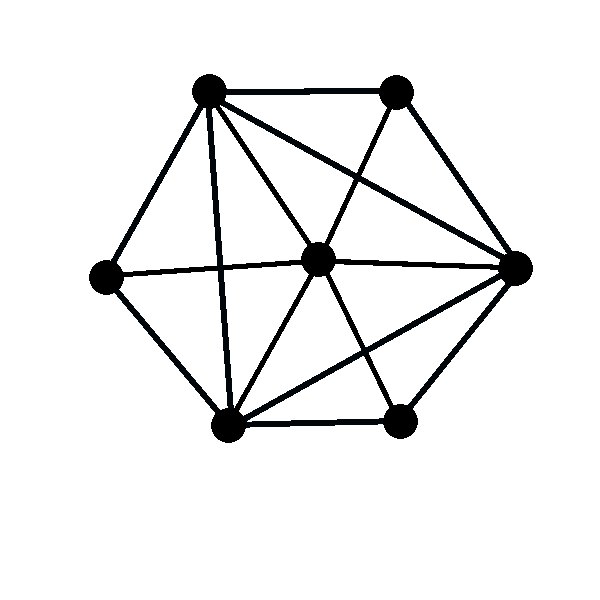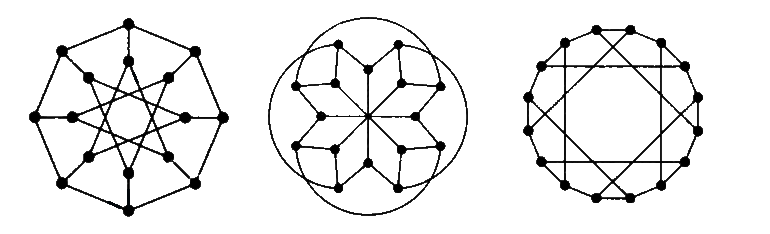MTH4140 Home

# MTH 4140: Midterm 1 Practice 1

Problem 1

Assume that $$A$$, $$B$$, and $$C$$ are three sets and that $$f:A\to B$$ and $$g:B\to C$$ are two functions. Consider the function $$h:A\to C$$ defined as $$h(x)=g(f(x))$$ for all $$x\in A$$. Prove that if $$h$$ is onto then $$g$$ is onto as well.

Problem 2

Determine the number of bijections $$f:\{1,2,3,4,5\}\to\{4,5,6,7,8\}$$ such that $$f(1)$$ is even.

Problem 3

Let $$A=\{1,2,3,\dots, n\}$$. Determine the number of binary relations on the set $$A$$ that are reflexive but not symmetric.

Problem 4

Assume that the sequence $$a_n$$ is defined as $$a_0=2$$, $$a_1=10$$, and $$a_{n+2}=10a_{n+1}-21a_n$$ for every $$n\geq 0$$. Use the principle of mathematical induction to prove that $$a_n=3^n+7^n$$ for all $$n\geq 0$$.

Problem 5

Let $$V=\{1,2,3,4,5\}$$ be the vertices of a graph $$G$$. Two vertices $$i$$ and $$j$$ are joined by an edge if and only if $$i+j$$ is odd. Draw the graph $$G$$.

Problem 6

Determine the complement of the graph shown in the picture below.Problem 7

Determine which of the pairs of graphs below are isomorphic.Problem 8

Assume that the graph $$G$$ can be drawn without lifting pen from the paper and without tracing the same line twice and that this drawing can be made in such a way that it starts and ends in the same vertex. Prove that the graph $$G$$ does not have cut edges.

Problem 9

Prove that for each integer $$k\geq 2$$ there exists a bipartite graph $$G$$ with $$2^k$$ vertices whose each vertex has degree $$k$$.

Problem 10

A graph is called red-green triangle free if all of its edges can be painted in red and green in such a way that there is neither a red triangle nor a green triangle. What is the maximal number $$e$$ of edges that a red-green triangle free graph can have, if the graph has $$6$$ vertices?